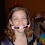## Monday, June 30, 2008

### Opening Day Jitters

A high school has a strange principal. On the first day, he has his students perform an odd opening day ceremony:

There are one thousand lockers and one thousand students in the school. The principal asks the first student to go to every locker and open it. Then he has the second student go to every second locker and close it. The third goes to every third locker and, if it is closed, he opens it, and if it is open, he closes it. The fourth student does this to every fourth locker, and so on. After the process is completed with the thousandth student, how many lockers are open?

1.31, which is the number of squares between 0 and 1000. For a locker number L, where A*B=L, the locker will be open and then shut in pairs (by the Ath and Bth students). In order to be open at the end of the process, the locker number has to have an odd number of factors, and the only way this can happen is if the number is a perfect square (A*A=L).

2.You've got it, Abe. The lockers that remain open are perfect squares. They are the only numbers divisible by an odd number of whole numbers; every factor other than the number's square root is paired up with another. Thus, these lockers will be "changed" an odd number of times, which means they will be left open. All the other numbers are divisible by an even number of factors and will consequently end up closed.

So the number of open lockers is the number of perfect squares less than or equal to one thousand. These numbers are one squared, two squared, three squared, four squared, and so on, up to thirty one squared. (Thirty two squared is greater than one thousand, and therefore out of range.) So the answer is thirty one.

Leave your answer or, if you want to post a question of your own, send me an e-mail. Look in the about section to find my e-mail address. If it's new, I'll post it soon.

Please don't leave spam or 'Awesome blog, come visit mine' messages. I'll delete them soon after.

Enter your Email and join hundreds of others who get their Question of the Day sent right to their mailbox

The Lamplight Manor Puzz 3-D